alonsoformula In SpanishWhat are they?  |  How are they named? If we know the formula  | If we know the name  |  Examples  |  Exercises

What are they?

They are compounds formed by a metal and hydrogen, in which the hydrogen has an oxidation number –1.

The hydrides of groups 1 and 2 are more ionic than covalent. The hydrides of groups 13 and 14 are more covalent than ionic. But they have the same name, except boron hydride which is named as H + nometal compound.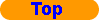How are they named?

The name is formed by two words. The first word is the name of the metal. The second word is the root of hydrogen plus the suffix "–ide"

METAL hydrideIf we know the formula

In the formula: Write the name of the metal followed by "hydride".If we know the name

In the name: Remember that you must know the symbols of the elements and the oxidation numbers which are invariable.

1. Write the symbol of the first element.
2. Write the symbol of the hydrogen.
3. The number of hydrogen matches up with the oxidation number of metal.Examples

 LiH Lithium hydride CaH2 Calcium hydride AlH3 Aluminium hydride GeH4 Germanium hydride SnH4 Tin hydrideExercises

In Formulae you have an exercise to write the names of these substances and to check your results. You also have the answer to the exercise.

In Names you have an exercise to write the formulae for these substances and to check your results. You have to introduce the formulae without subscripts, for example for water = H2O. You also have the answer to the exercise.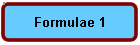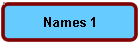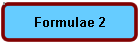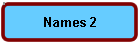Home | Introduction | Oxidation number | Mechanics | Kind of substances | Elementary substances | Metal oxides | Nonmetal oxides | Metal-nonmetal compounds | Nonmetal-nonmetal compounds | Metal hydrides | Hydracids | H with nonmetal | Hydroxides | Oxyacids | Oxysalts | Acid salts | Exercises | Periodic Table | Links
Author: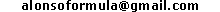WWW.ALONSOFORMULA.COM Formulación Inorgánica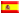Formulación OrgánicaFormulación Inorgánica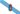Formulación OrgánicaFormulació InorgánicaFormulació OrgánicaEzorganikoaren Formulazioa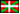Nomenclature of Inorganic Q.Física y Química de ESOFísica e Química de ESOFQ de 1º de BachilleratoFQ de 1º de BacharelatoQuímica de 2º de BachilleratoPrácticas de Química